• 0

# Hypothesis Testing

Go to solution Solved by Rupinder N,

Hypothesis Testing

Hypothesis Testing - it is the process of using statistical tests to determine if the observed differences between two or more samples is statistically significant or not. A null hypothesis (Ho) is a stated assumption that there is no difference or the difference is due to a random chance while the alternate hypothesis (Ha) is a statement that there is a true difference. With the help of hypothesis testing, we arrive at one of the following conclusions.

1. Fail to reject the Null hypothesis (accept the Null hypothesis)
2. Reject the Null hypothesis (accept the Alternate hypothesis)

From a practical point of view, hypothesis testing allows to collect sample sizes and make decisions based on facts and it takes away the decisions based on gut feeling or experience or common sense. You have statistical proof of whatever you "feel" or "think" is right.

An application oriented question on the topic along with responses can be seen below. The best answer was provided by Rupinder N on 22nd September 2017.

## Question

Q 13. Hypothesis testing is one of the powerful methods used in Six Sigma methodology. In the pursuit of excellence, how important is hypothesis testing? In which phases of an improvement project, is it likely to be used?

Note for website visitors - Two questions are asked every week on this platform. One on Tuesday and the other on Friday.

## Recommended Posts

• 0
• Solution

Hypothesis Testing is among the most powerful tools used in Business Excellence. It takes away the decisions based on gut feeling or experience or common sense e.g. Site A has better performance than Site B, we should hire more experienced employees as their accuracy is higher, it takes lesser time if we use System A vs System B, older customers are less likely to use self-help as compared to other age groups, are we meeting the cut-off defective %age or not, based on the proportion defectives we see. Hypothesis testing allows to collect valid sample sizes and make decisions for population - it keeps the gut feeling and statements such as "in our experience" out of the picture. You have statistical proof of whatever you "feel" or "think" is right.

What must be kept in mind is that it is an OFAT testing technique - only one factor under consideration can be varied while all other Xs must be maintained constant.

Hypothesis Testing can be used in any and every phase of the DMAIC cycle.

Define - Usually all "1" tests or tests where we compare a population to an external standard are used in this phase e.g. 1 proportion test (if I have x out of y defects, am I meeting the client quality target of 95%?), 1 Sample Z, 1 Sample T, 1 Sample Sign etc. (Has the cost of living gone up as compared to the mean or median cost 10 years ago?). It helps us decide "do we even have a problem".

Measure - One can look at data and the eye can catch a "trend". But can we really say that the performance has dipped, is the difference in performance statistically significant. Hypothesis testing can give you the answer.

Analyze - this hardly needs any explanation as everyone has using hypothesis testing extensively in this phase to compare two populations or multiple populations e.g. do the five swimming schools create the same proportion of champions out of all enrolled in them, is the lead time for a process on machine A better than machine B, does Raw Material X give better quality than Raw Material Y, does Training Methodology 1 give better results as compared to Methodology 2, 3 and 4, does Vendor A have fewer billing discrepancies than Vendor B etc.

Improve - tests involving two populations are generally used.  E.g. comparing Y pre and post solution implementation (we implemented a solution to improve the yield of a machine). Is the post-solution yield higher than pre-solution yield, is the TAT post solution better than the TAT before implementing the solution, are more customers buying our product than before etc.

Control - We get different CTQ numbers every month post we made an initial improvement. Can we really say that we have improved as compared to before? For 5 months after improve, if we saw a lower number for the metric, was that really different than other months. Can we say that we are consistent? We can use Hypothesis testing again.

Business Excellence is nothing but an iterative process to drive excellence throughout the business. As Hypothesis Testing helps us validate or invalidate what we suspect every step of the way in the DMAIC cycle, it is a "must use" tool for the armor.

##### Share on other sites
• 2

Hypothesis testing is an essential procedure in statistics , it evaluates two mutually exclusive statements about a population to determine which statement is best supported by data i.e which statement is statistically significant. But, why do we need hypothesis testing ? that is because we are making our conclusion about a population basis the sample data – hypothesis tests helps assess the likelihood of this possibility that the sample data is representative of the population data. This itself makes hypothesis testing very significant as it helps us assess the population parameters using the sample statistics.

Hypothesis tests can be used across Define, Measure, Analyze and Improve phase of an improvement project

Define Phase : To test whether the target set is significantly different from the baseline performance.

Measure phase : Understanding the likelihood that a data sample comes from a population that follows a given probability distribution (i.e. normal, exponential, uniform, etc.)

Analyse Phase : For screening potential causes. Evaluating several process factors (process inputs, or x’s) in a designed experiment to understand which factors are significant to a given output, and which are not.

Improve phase :  Evaluating a proposed process improvement, using pilot study output, to see if its effect is statistically significant, or if the same improvement could have occurred by random chance.

Thanks

Jisha Nair

##### Share on other sites
• 1

A hypothesis test is a statistical test that is used to determine whether there is enough evidence in a sample of data to infer that a certain condition is true for the entire population.

A hypothesis test examines two opposing hypotheses about a population: the null hypothesis and the alternative hypothesis. The null hypothesis is the statement being tested. Usually, the null hypothesis is a statement of "no effect" or "no difference". The alternative hypothesis is the statement you want to be able to conclude is true.

Based on the sample data, the test determines whether to reject the null hypothesis. You use a p-value, to make the determination. If the p-value is less than or equal to the level of significance, which is a cut-off point that you define, then you can reject the null hypothesis.

A common misconception is that statistical hypothesis tests are designed to select the more likely of two hypotheses. Instead, a test will remain with the null hypothesis until there is enough evidence (data) to support the alternative hypothesis.

Hypothesis testing is used in the Six Sigma Analyze Phase for screening potential causes.

All hypotheses are tested using a four-step process. The first step is for the analyst to state the two hypotheses so that only one can be right. The next step is to formulate an analysis plan, which outlines how the data will be evaluated. The third step is to carry out the plan and physically analyze the sample data. The fourth and final step is to analyze the results and either accept or reject the null. Try to re-run the test (if practical) to further confirm results. The next step is to take the statistical results and translate it into a practical solution.

It is also possible to determine the critical value of the test and use to calculated test statistics to determine the results. Either way, using the p-value approach or critical value should provide the same result. There could be a problem with centering where the process is not centered, it may be precise but not accurate. Processes may be accurate but not precise.
Data collection establishes the foundation for appraising the quality of a product or service. But without correct data processing, it becomes challenging to make an objective conclusion. Sometimes, the observation is wrongly interpreted. This is the hypothesis to be tested.

Essentially good hypotheses lead decision-makers to new and better ways to achieve your business goals. When you need to make decisions such as how much you should spend on advertising or what effect a price increase will have your customer base, it’s easy to make wild assumptions or get lost in analysis paralysis. A business hypothesis solves this problem, because, at the start, it’s based on some foundational information Theory tells you what you can generally expect from a certain line of inquiry. A hypothesis based on years of business research in a particular area, then, helps you focus, define and appropriately direct your research. You won’t go on a wild goose chase to prove or disprove it. A hypothesis predicts the relationship between two variables. If you want to study pricing and customer loyalty, you won’t waste your time and resources studying tangential areas.

##### Share on other sites
• 1

A hypothesis is an educated guess about something in the world around you. It should be testable, either by experiment or observation. For example:

• A new medicine you think might work.
• A way of teaching you think might be better.
• A possible location of new species.
• A fairer way to administer standardized tests.

It can really be anything at all as long as you can put it to the test.

## What is a Hypothesis Statement?

If you are going to propose a hypothesis, it’s customary to write a statement. Your statement will look like this:
“If I…(do this to an independent variable)….then (this will happen to the dependent variable).”
For example:

• If I (decrease the amount of water given to herbs) then (the herbs will increase in size).
• If I (give patients counseling in addition to medication) then (their overall depression scale will decrease).
• If I (give exams at noon instead of 7) then (student test scores will improve).
• If I (look in this certain location) then (I am more likely to find new species).

A good hypothesis statement should:

• Include an “if” and “then” statement (according to the University of California).
• Include both the independent and dependent variables.
• Be testable by experiment, survey or other scientifically sound technique.
• Be based on information in prior research (either yours or someone else’s).
• Have design criteria (for engineering or programming projects).

## What is Hypothesis Testing?

Hypothesis testing in statistics is a way for you to test the results of a survey or experiment to see if you have meaningful results. You’re basically testing whether your results are valid by figuring out the odds that your results have happened by chance. If your results may have happened by chance, the experiment won’t be repeatable and so has little use.

Hypothesis testing can be one of the most confusing aspects for students, mostly because before you can even perform a test, you have to know what your null hypothesis is. Often, those tricky word problems that you are faced with can be difficult to decipher. But it’s easier than you think; all you need to do is:

1. Figure out your null hypothesis,
3. Choose what kind of test you need to perform,
4. Either accept or reject the null hypothesis.

What is the Null Hypothesis?

If you trace back the history of science, the null hypothesis is always the accepted fact. Simple examples of null hypotheses that are generally accepted as being true are:

1. DNA is shaped like a double helix.
2. There are 8 planets in the solar system (excluding Pluto).
3. Taking Vioxx can increase your risk of heart problems (a drug now taken off the market).

How do I State the Null Hypothesis?

You won’t be required to actually perform a real experiment or survey in elementary statistics (or even disprove a fact like “Pluto is a planet”!), so you’ll be given word problems from real-life situations. You’ll need to figure out what your hypothesis is from the problem. This can be a little trickier than just figuring out what the accepted fact is. With word problems, you are looking to find a fact that is nullifiable (i.e. something you can reject).

Hypothesis Testing Examples #1: Basic Example

A researcher thinks that if knee surgery patients go to physical therapy twice a week (instead of 3 times), their recovery period will be longer. Average recovery times for knee surgery patients is 8.2 weeks.

The hypothesis statement in this question is that the researcher believes the average recovery time is more than 8.2 weeks. It can be written in mathematical terms as:

H1: μ > 8.2

Next, you’ll need to state the null hypothesis (See: How to state the null hypothesis). That’s what will happen if the researcher is wrong. In the above example, if the researcher is wrong then the recovery time is less than or equal to 8.2 weeks. In math, that’s:

H0 μ ≤ 8.2

Rejecting the null hypothesis:

Ten or so years ago, we believed that there were 9 planets in the solar system. Pluto was demoted as a planet in 2006. The null hypothesis of “Pluto is a planet” was replaced by “Pluto is not a planet.” Of course, rejecting the null hypothesis isn’t always that easy — the hard part is usually figuring out what your null hypothesis is in the first place.

Hypothesis testing categorization:

Hypothesis testing is categorized as a parametric test and nonparametric test. The parametric test includes z-test, t-test, f-Test and x2 test. The nonparametric test includes sign test, Wilcoxon Rank-sum test, Kruskal-Wallis test and permutation test.

Parametric test:  In this test, samples are taken from a population with known distribution (normal distribution), and a test of population parameters is executed.

Nonparametric test: Also called distribution-free test, this test does not require the population to conform to a normal distribution, nor do the popular parameters need to be statistically estimated.

How important is hypothesis testing?

People refer to a trial solution to a problem as a hypothesis often called an "educated guess" because it provides a suggested solution based on the evidence. However, some scientists reject the term "educated guess" as incorrect. Experimenters may test and reject several hypotheses before solving the problem. Much of running a small business is a gamble, buoyed by boldness, intuition, and guts. But wise business leaders also conduct formal and informal research to inform their business decisions.

“Good research starts with a good hypothesis”, which is simply a statement making a prediction based on a set of observations.

The purpose of hypothesis testing is to make a decision in the face of uncertainty. We do not have a fool-proof method for doing this: Errors can be made.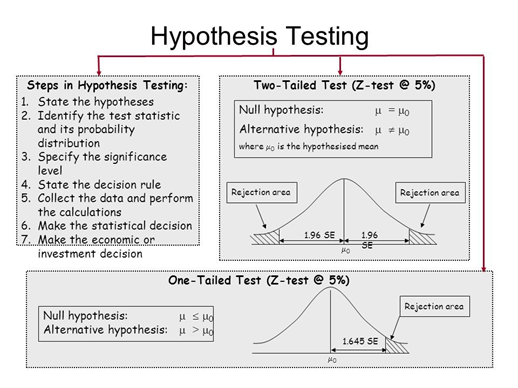In which phases of an improvement project, is it likely to be used?

It is used in analyze phase of the DMAIC improvement cycle. Critical X’s are identified and to measure the effect of each X on Y, the hypothesis testing is used in Analyze phase.

##### Share on other sites
• 0

Primarily hypothesis is used in Analysis of the problem area to screen potential causes. Hypothesis test helps assess if proposed improvement project results in statistically significant improvement. In case of analysis of various process factors, hypothesis test helps to assess which all factors have significant influence on outcome. It also helps to figure out if the improvement observed in process is due to the actions initiated by us or due to chance variation.

Hypothesis testing helps to address the possible sampling error involved in the study of sample data of the population. Overall in pursuit of excellence hypothesis test helps to validate any possible assumptions involved in the analysis and cut down on possible errors due to assumptions.

##### Share on other sites
• 0

Hypothesis test is a statistical techniques which is used to evaluate two mutually exclusive statements about a population to determine which statement is best supported by the sample data. Hypothesis testing helps in taking a decision in the face of uncertainty.

Hypothesis testing is helpful in taking decisions in a wide variety of areas. For example, in case of a business one can use the technique to determine whether customer experience has a significant bearing on sales or is it the price which is more significant. This can help the owner of the business to pay more attention to the pain area and take corrective measures.

Under the DMAIC framework, Hypothesis Testing is used in the Analyse Phase. It helps in prioritizing the X's so that solutions and recommendations can be provided to correct and improve the significant X's. Two-sample t-test and Chi-square are most commonly used hypothesis tests in Six Sigma work

##### Share on other sites
• 0

Hypothesis testing is an objective method of making decisions or inferences from sample data. Typically carried out by comparing what we have observed to what we have expected and if one of the statements was true.

These are the requirements for the test:

Null statement or Ho is an assumption about the population from the sample data used to decide whether it is true or otherwise. Also known as skeptic’s hypothesis it is sticking to the status quo of the case. Alternative hypothesis on the other hand is a researcher’s question to the claim.

Since we are inferring the population from a sample one needs to also asses the risk involved of being wrong.

Significance level is the probability of rejecting the null hypothesis when it is true. Also known as type I error. This is normally set at 5% (0.05). Test statistic is the value calculated from a sample to decide whether to accept or reject null hypothesis.

p Value is the probability of obtaining a test statistics.

If p is less than alpha=5%, the null hypothesis is rejected at 5% significance level and the test result is called “statistically significant”.

If p is less than alpha=1%, the null hypothesis is rejected at 1% significance level and the test result is called “highly significant”.

Test statistic = [Relevant Statistics - Hypothesized Parameter]/Standard Error of Relevant Statistic.

= [Observed-Expected]/Standard Error

Based on the awareness of Standard deviation one needs to use either z test or t test. And the table below shows the relationship of the choice made based on the statistical result.

 DECISION Ho TRUE Ho FALSE Do not Reject Ho No Error  ( 1-alpha) Type II Error (beta) Reject Ho Type I Error (alpha) No Error (1-beta)

Predominantly used during the analyse phase of the DMAIC roadmap. Below are the criteria one needs to be familiar with to have a successful test and subsequent effective prediction.

•  It would be wonderful if we could force both alpha and beta to equal zero.  Unfortunately, these quantities have an inverse relationship.  As alpha increases, beta decreases and vice versa.
•  alpha is the producer’s risk in an experiment with a new drug or the skeptics leeway to the status quo and beta, the consumer’s risk to the new drug or declined researcher’s claim. Both are to assessed and determined based on the potential loss and the severity of a decision.
• The only way to decrease both alpha and beta is to increase the sample size.  To make both quantities equal zero, the sample size would have to be infinite—you would have to sample the entire population.
• Power of the test (1– beta) is the probability of correctly rejecting the false null hypothesis. This can be enhanced when the variation in the sample is reduced, increase in the sample size and an increase in alpha.
• For parametric tests, the statistical power is higher and it helps one to significantly detect an effect.
##### Share on other sites
• 0

A Hypothesis is basically a thought or belief that a particular input might affect an output.Hypothesis Testing is a systematic approach of testing this belief statistically and deciding whether the hypothesis can be retained as reasonable and realistic or should be rejected as impractical or insupportable. A hypothesis is developed for the population and statistical tests are used to determine whether there is enough evidence in a sample of data (representing the population) to infer that a certain condition is true for the entire population.

A Six Sigma Project is implemented using the DMAIC or DMADV Methodology.

• DMAIC: Define -> Measure -> Analyze -> Improve -> Control
• DMADV: Define -> Measure -> Analyze -> Design -> Verify

Hypothesis testing is used in the Analyze phase. In this phase, potential Xs (Inputs) which might have a bearing on the defined Y (output) of the six sigma projects are listed.  Out of the potential Xs, critical Xs, which are expected to affect the output the most, are identified and checked if the identified critical Xs are sufficient to improve the Output.

An hypothesis test will help the team in cases like determining if a modified process B has “significantly” improved yield when compared to the original process A or if a quality parameter is independent of a given production condition and so on.

##### Share on other sites
• 0

Answer 2 from Excellence Club 66:  Hypothesis testing

In a process, we may face Problem with Centering and/or Problem with Spread. Below diagram will allow us to understand these two problems in detail: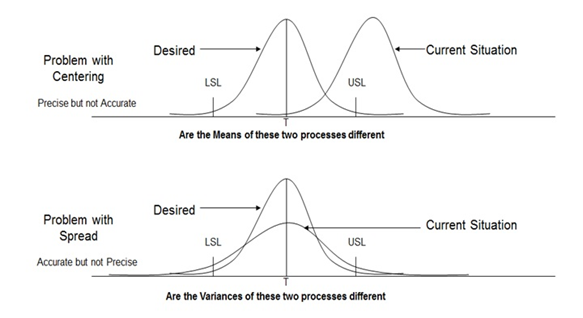Practical Six Sigma Problems that require Hypothesis Testing

Hypothesis testing tells us whether there exists statistically significant difference between the data sets for us to consider that they represent different distributions.

What is the difference that can be detected using Hypothesis Testing?
For Continuous data, hypothesis testing can detect Difference in Average and Difference in Variance. For Discrete data, hypothesis testing can detect Difference in Proportion Defective.

Steps in Hypothesis Testing:

• Step 1: Determine appropriate Hypothesis test
• Step 2: State the Null Hypothesis Ho and Alternate Hypothesis Ha
• Step 3: Calculate Test Statistics / P-value against table value of test statistic
• Step 4: Interpret results – Accept or reject Ho

Mechanism:

• Ho = Null Hypothesis – There is No statistically significant difference between the two groups
• Ha = Alternate Hypothesis – There is statistically significant difference between the two groups

Hypothesis Testing Errors:

Type I Error – P (Reject Ho when Ho is true) = α
In type I Error, we reject the Null Hypothesis when it is true. It is also called as Alpha error or Producer’s Risk.

Type II Error - P (Accept Ho when Ho is false) = β
Similarly, in type II Error, we accept Null Hypothesis when it is false. It is also called as Beta error or Consumer’s Risk.

Six Sigma Hypothesis Testing Errors

P Value – Also known as Probability value, it is a statistical measure which indicates the probability of making an α error. The value ranges between 0 and 1. We normally work with 5% alpha risk, a p value lower than 0.05 means that we reject the Null hypothesis and accept alternate hypothesis.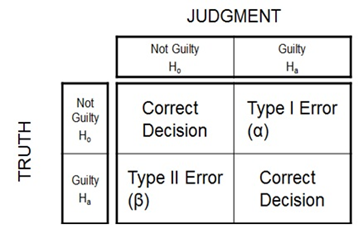Types of Hypothesis Testing:
We use the following grid to select the appropriate hypothesis test depending on the data types: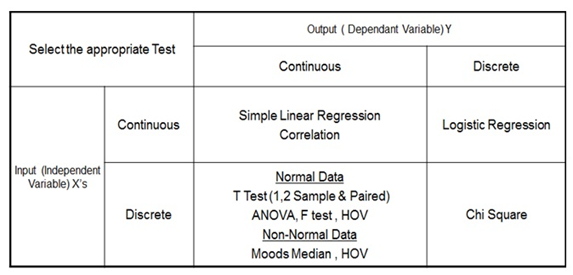Types of Six Sigma Hypothesis Testing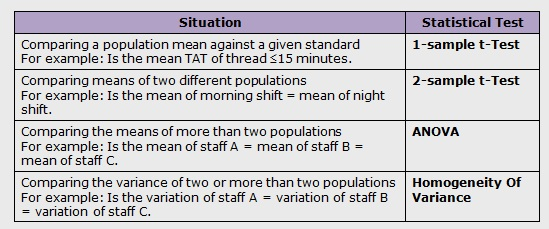Normal Continuous Y and Discrete X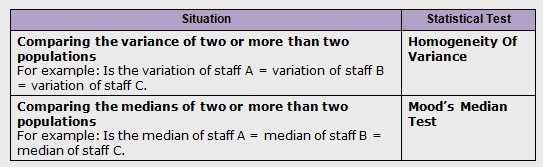Non-Normal Continuous Y and Discrete X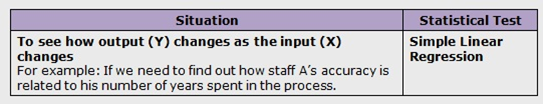Continuous Y and Continuous X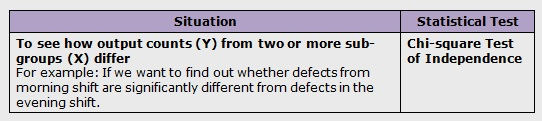Discrete Y and Discrete X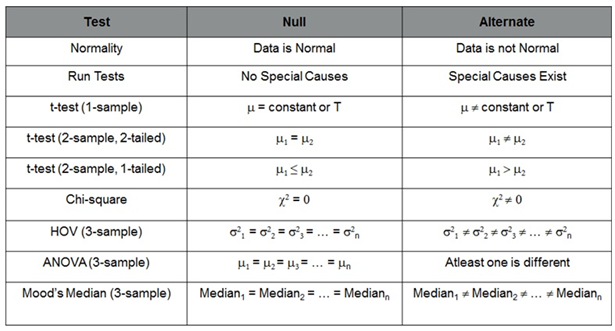Six Sigma Hypothesis Test – Null and Alternate Summary

How important is hypothesis testing?

The purpose of hypothesis testing is to make a decision in the face of uncertainty. We do not have a fool-proof method for doing this: Errors can be made.

In which phases of an improvement project, is it likely to be used?

It is used in analyze phase of the DMAIC improvement cycle. Critical X’s are identified and to measure the effect of each X on Y, the hypothesis testing is used in Analyze phase.

##### Share on other sites
• 0

Hypothesis testing is used in ANALYZE PHASE for testing potential causes. The probability that the data samples actually came from the same underlying population can be calculated. If this p-value is less than 0.05 than we conclude that the two samples likely came from different underlying populations.

Example: p-value= 0.02; means only 2% chance that data samples came from the same underlying population.

It assists us in problem-solving:

1. Evaluating a proposed process improvement to see if its effect is statistically significant, or if the same improvement could have occurred by random chance.
2. Evaluating several process factors (x's) in a designed experiment to understand which factors are significant to a given output and which are not.
3. To understand the data samples follows which given probability- normal, exponential, uniform, etc

Example:  An individual working to improve his vehicle's fuel economy might run a hypothesis test comparing fuel economy at driving speeds of 60 mph and 70 mph on a highway.

The result might show that driving at the lower speed has a statistically significant effect on the CTQ, win the case is miles-per-gallon fuel economy. The actual improvement in fuel economy might only be 0.5 miles per gallon, which be deemed not worth the extra time it will take to get work each day.

##### Share on other sites
• 0

Hypothesis testing is an important statistical tool that helps us to take an objective decision about a population parameter based on a reasonably small sample. Popular decisions made are whether:

• The mean value of the population can be considered equal, more or less than a reference value.
• The means of two populations may be considered equal or not.
• The variance of a population is equal to a reference value
• The variance between two populations may be considered equal

The test of hypothesis (TOH) will be useful during the Analyze phase of DMAIC cycle. Once we have short listed a set of potential cause factors (x factors) based on a funneling method, say, a subjective rating method, TOH helps us to to narrow down to factors that have a significant influence on the outcome, in an objective manner. The TOH methods offer a good range of choices on the type of tool to be selected for a given data type, whether it is variable, discrete, standard deviation known or unknown, parametric, non-parametric, paired test etc.

TOH is also the fundamental principle upon which other advanced statistical tools such as ANOVA (Analysis of Variance), DOE (Design of Experiments) are evolved. Thus a knowledge and understanding of TOH is very essential to enhance one's competence to use several other tools as part of the Six Sigma cycle.

Apart from the Analyze phase, during the Improve phase, once we identify multiple solutions, TOH or TOH based tools may be found useful for determining the relative effectiveness of the solutions as well.

The versatility of TOH to adapt to different types of data and its ability to help derive conclusions by using very limited samples, with the known confidence levels, makes it an indispensable tool in the pursuit for excellence. It may also be noted that the complexities associated in handling the statistical calculations have been greatly simplified and made very user friendly by modern software applications.

##### Share on other sites
• 0

In my humble opinion, Hypothesis Testing (HT) would be the life of the Six Sigma Methodology, where reality checks are conducted to validate one way or the other, assumptions, hunches or expert opinion. In short, Hypothesis Testing is the password to access the Domain of Excellence. Of course, access to the domain alone is not sufficient to achieve excellence. However, HT provides the opportunity for the same.

HT symbolises the value add, the Six Sigma methodology brings to the table when compared with traditional improvement approaches. HT captures the essence of the "Data driven-ness" of the Six Sigma Methodology. In a validated Measurement System, HT remains the ultimate decision enabler.

Apart from the uses of HT during the Six Sigma project life-cycle, HT is very effective even when used as a stand-alone, "Six Sigma Infrastructural process tool". Any comparisons of period based performance becomes that much enriched with HT. Daily, Weekly, Monthly Performance reports all become even more smarter when combined with HT. All staff can take more educated decisions in their daily work if they apply HT.

In the Analyse Phase, HT is used to identify the Vital few Xs from the overall X universe. Further in the Improve Phase, HT is used to validate the results of the pilots. Again, after full blown implementation of the improvements in the Control Phase, HT is again used to validate the success of the project.

Apart from the above-mentioned, almost mandatory uses of HT, in certain very specific situations, HT is sometimes also used in the Measure Phase to come to a conclusion on the Measurement System. In some even more peculiar situations, HT can come in handy in Project selection during the Define Phase.

##### Share on other sites
• 0

Hypothesis Testing is used in the Analyze phase. Hypothesis testing tells us whether there exists statistically significant difference between the data sets for us to consider that they represent different distributions.

For Continuous data, hypothesis testing can detect Difference in Average and Difference in Variance. For Discrete data, hypothesis testing can detect Difference in Proportion Defective. To Summarize the detail in pictorial form is given below.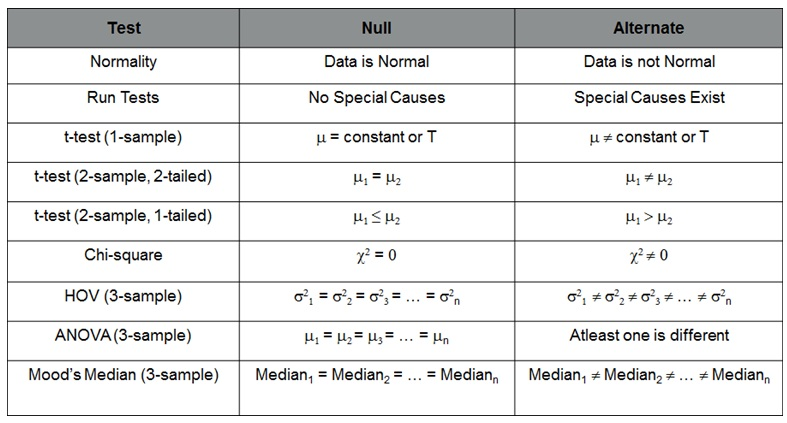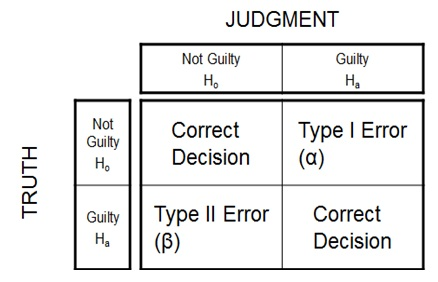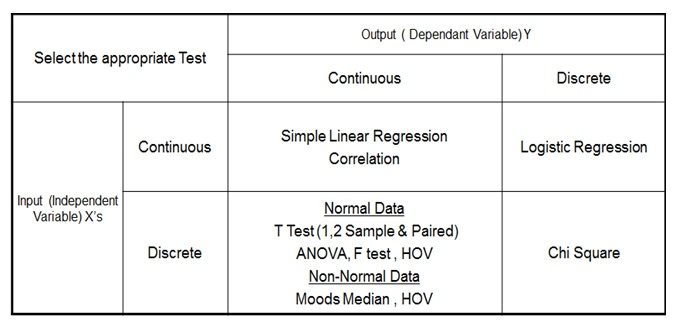##### Share on other sites
• 0

Generally the good hypothesis decisions leads to new understandings and better alternatives to achieve the goals . Lets take an example as an organization we decide to spend on advertizing or what effect will result in price hike on customers. Its easy tobmake a wild assumptions.

A business hypothesis always helps to solve the problems . In the start it takes support of some foundational information. Basically, hypothesis is theory. The theory details you generally expect from a certaion thought of enquiry.

A hypothesis based on years of business research in a particular field hepls you to focus ,define and appropriately direct your research.

You will not blindly or with a gut f÷ling chase to approve or disapprove it.

The hypothesis predicts the relationship between two variables. Data collection establishes the foundation for appraising the quality of a product or service.but without correct data processing, it becomes challenging make an objective conclusion.it leads to wrong interpretation. Hypothesis testing is the process of using statistics to determine the probability that a specific hypothesis is true.

Hypothesis test evaluates two mutually exclusive statements about a population to determine which statement is bestvsupported by sample data.

Hypothesis testing is categorized as a parametric test and nonparametric test. The parametric includes z-test, t-test, and f-test.

The nonparametric test includes sign test, Wilcoxon rank sum test, Kruskal Wallis test and permutation test. Its uses in analyze phase

Examples:

The statement of problem

Suppose that the doctor claims those who are 17 yearscold have an average body temperature that is hogher than the commonly acepted human temperature of 98.6 degrees F .A simple random statistical samplevof 25 people ,each of age 17 is selected.The average temperature of the sample of the sample is found to be 98.9 degrees f .Further suppose that we know that the population standard deviation of every one who is 17 years old is 0.6 degress.

The null hypothesis

The claimbbeing investigated is that the average body temperature of everyone who is 17 yrs is greatervthan 98.6 degrees.This correspondes to the ststement x>98.6. The negation of this is that the population average is not greatervthan 98.6degress.In other words , the average temperature is les than or equal to 98.6 degrees.

I.e x<=98.6.

One of these statements must become the null hypothesis, and the other should be the altenative hypothesis. The null hypothesis contains equality. So for above, the null hypothesis Ho : x= 98.6.

Its a common practise to only state the null hypothesis in terms of an equals sign and not a greater or equal or less thannequal to.

The orther which does not contain equality is alternative hypothesis.

One or two tails.

The statement of our problem will determine which kind of test to use .if the alternative hypothesis contains a not equal to sign thenbwe use two tailed test.

In other two cases whenbthebaltrnative hypothesisbcontains strict inequality webuse one tailes test.

Choice ofba significance

Here we choose the valuebof alpha , our significance level.It is typical to let alpha be 0.05 or 0.01.For this example we will use 5% level.i.e the alpha = 0.05.

Choice of test statistic and distribution.

Now we need to determine which distribution to use .the sample is from a population that is normally distributed as the bell curve, so we can use the standard normal distribution. A table of z scores will be necessary.

The test statistic is found by the formulae for the mean of a sample, rather than thevstandard deviation we use standard error of the sample mean.Here n= 25 which hasba square root of 5. So the standard error is a 0.6/5 = 0.12.our test statistic is z = (98.9-98.6)/.12 = 2.5.

Accepting and Rejecting

At a 5% significance level, the critical value for a one tailed test is found from the table of z scores to be 1.645

Since the test statstic does not fall within the critical region we reject thevnull hypothesis.

The pvalue method.

There is a slight variation if we conduct our test using p values.

Here we see that a zscore of 2.5 has a p value of 0.0062.Since this less than the significance level of 0.05 we reject null hypothesis.

Conclusion

We conclude by stating the results of our hypothesis test.

The statistical evidence shows that eitherva rare event has occured or that the average temperature of thosevwho are 17yrs is greater than 98.6degrees.

##### Share on other sites
• 0

Hypothesis test evaluates two mutually exclusive statements about a population to determine which statement is best supported by the sample data. Research without hypothesis testing is like a sailor in the sea without compass. Hypothesis testing is used in Analyze phase in an improvement project.

##### Share on other sites
• 0

What is Hypothesis Testing:

To determine if the observed differences in the given samples are due to random chance or due to true differences in the samples, through statistical means

When it's used and why it is important:

Hypothesis Testing is used in the analyze phase of an improvement project (DMAIC).   It is extremely important and helps in making business decisions through statistical means. Let us see how it's achieved.

As we see from the equation, Y=f(x), vital factors that would impact the outcome, need to be scrutinized. There may be some instances where tools like Pareto chart might be able to highlight which of these factors are important. But sometimes these tools may not be able to determine the vital factors which can affect the outcome. This is where Hypothesis testing comes into the picture.

With hypothesis testing, we would be able to highlight the vital factors with relative ease. Also with it, we would be able to tell statistically which can lend support to your business decisions.

Let us speak with an example:

To find the mileage of a car, we have a null hypothesis (H0) which says that tyre pressure, fuel and speed determine the car mileage.  Let us do a Design of Experiment (DOE) for this.

Car Mileage:

 Factors Tyre Pressure Fuel Speed Factor1 20~25 psi Gasoline/Diesel 40~50 km/hr Factor2 30~35 psi Gasoline/Diesel 50~60 km/hr Factor3 30~35 psi Gasoline/Diesel 40~50 km/hr Factor4 30~35 psi Gasoline/Diesel 60~70 km/hr

It is observed that factor3 (in bold) serves the optimal value for the mileage of a car. Therefore, our null hypothesis of tyre pressure, fuel and speed determine the car mileage.  There may be instances where even when a hypothesis is proven true, it may not statistically add much difference, but which can lend support to business decisions.

Conclusion:

Thus we can see how important is hypothesis testing.

##### Share on other sites
• 0

The Hypothesis testing  is tool used in inferential statistics.Hypothesis tells us whether there  exists statistically significant difference between the data set to consider that they represents different distributions.Here a null hypothesis and an alternative hypothesis are stated.The null hypothesis is a statement about the value of a population parameter such as mean,and must contain the condition of equality.The alternate hypothesis is a statement that must be true if null hypothesis is false.A null hypothesis can only be rejected or fail to be rejected,it cannot be accepted because of a lack of evidence to reject it. The Hypothesis test is used in ANALYZE phase of six Sigma methodology.The practical problem was created in earlier phases.The Hypothesis test reviews the families of variation statistically to determine the significant contributors to the output.The steps involved are: State the null hypothesis (Ho) and alternative hypothesis (Ha).Choose the level of significance (alpha). Determine the rejection region for the statistics of interest.Calculate the test statistic.Decide whether the null hypothesis should be rejected.State the conclusion in terms of the original problem.

##### Share on other sites
• 0

In hypothesis testing, the statistician is likely to prove that whether the data is statistically significant and unlikely to have occurred by chance alone. A hypothesis test is a test of significance.

Once the statistician has collected data and you test hypothesis against the likelihood of chance, he draws his final conclusion. If he reject the null hypothesis than he is claiming that the result is statistically significant and that it did not happen by luck or chance. As such, the outcome proves the alternative hypothesis. If he fails to reject the null hypothesis than it is concluded that you did not find an effect or difference in your study.

Hypothesis testing is used in the six sigma Analyse phase for screening potential causes.

##### Share on other sites
• 0

Decision making is very crucial in every business and are taken based on experience, assumptions, judgment.

Most of the time businesses are trying to arrive at a conclusion for below questions:

1. Is the new design proposed is more robust?
2. Is the new process is cost-effective, increase the productivity, improve the performance?
3. Does adding new features to the product will increase the sale?

Mostly decisions for the above questions are made on judgement. Accuracy of the judgement is based on data. Statistical methods will help us take data based decision.

After a problem is identified, then a make a hypothesis about what will happen during the testing. In other words, the purpose of the hypothesis is a proposed solution to the problem.

Hypothesis cannot be proven correct from the data obtain in the experiment, instead hypothesis are either supported by the data collected or refuted by the data collected.

Hypothesis is used in analyze phase: It helps develop a criteria (educated guess) to accept or reject  a decision based on data obtained from experiments.

Hypothesis Testing Errors:

Type I Error – P (Reject Ho when Ho is true) = Alpha

Type II Error – P (Accept Ho when Ho is false) = Beta

Confidence Level – The probability of correctly accepting Ho

Power of Test – The probability of correctly rejecting Ho

 Reality Ho is true Ho is false Decision Ho not rejected Correct Decision (confidence = 1- alpha) Type II error (Beta Risk) Ho is rejected Type I error (Alpha Risk) Correct Decision (Power = 1- Beta)

##### Share on other sitesThis topic is now closed to further replies.
• ### Who's Online (See full list)

There are no registered users currently online

• ### Forum Statistics

• Total Topics
2,868
• Total Posts
14,517
• ### Member Statistics

• Total Members
55,057
• Most Online
888

×
×
• Create New...